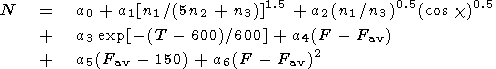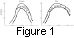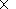International Journal of Geomagnetism and Aeronomy
Vol 2, No. 2, August 2000

# Regular variations of the electron density at 170 km

L. A. Shchepkin, G. P. Kushnarenko, and G. M. Kuznetsova

Institute of Solar-Terrestrial Physics, Irkutsk, Russia

I. A. Freizon

Institute of Terrestrial Magnetism, Ionosphere, and Radio Wave Propagation, Troitsk, Moscow Region, Russia

### Abstract

The regular variations of the electron density at the 170-km height are studied on the basis of the model simulation. This problem is of a certain interest for studying the middle ionosphere to improve the International Reference Ionospheric (IRI) model. For the calculations we used the model based on the experimental data set with the help of the semiempirical regression equation, which relates the values of N with the thermosphere characteristics. The electron density values modeled at 170 km are compared with IRI.

### 1. Introduction

At the IRI Workshop in 1996 it was formulated that [Bilitza, 1997b, p. 3] "The future task is: to establish an f170 model in terms of solar zenith angle, latitude, solar sunspot number and season." The 170-km level lies at the region of the F1 ledge. There is a minimum variability of electron density near this level [Zhang and Radicella, 1996].

The first step in the frame of this task is to study the main regularities in variations of N170 (or the plasma frequence f170). This may be done with the help of the semiempirical model describing the N170 dependence on the thermospheric characteristics [Shchepkin et al., 1997]. Using the MSIS 86 model [Hedin, 1987] we can evaluate the N170 dependence on latitude, longitude, solar activity indices, geomagnetic disturbances, day of the year, and local time (or solar zenith angle.)

The semiempirical model used [Shchepkin et al., 1997] was based on the experimental data on N at 120-200 km with the help of the regression equation which describes the N relation to the thermospheric gas characteristics.

A comparison of the model calculations with the latest version of the International Reference Ionosphere (IRI 95) [Bilitza, 1997a] is made.

### 2. The Model

The analytical expression, which describes the relation of N with the neutral gas characteristics, was obtained from the analysis of the N(h) profiles calculated with the help of the aeronomical model of the type, presented by Vinitsky et al. . We used this expression as a regression equation:(1)

Here n1 is the atomic oxygen concentration at 120 km, n2 and n3 are the O 2 and N 2 concentrations, respectively, T is the exospheric temperature, F is the 10.7-cm solar flux, Fav is the value of F averaged over the 81-day period, and c is the solar zenith angle.

In (1) the term with the a1 coefficient describes the N dependence on the relation between the atomic oxygen and molecular densities. The next term describes the dependence on cos c and the relative atomic oxygen content. The temperature term (with the a3 coefficient) shows the N response to the variation of the particle column content related to the T variations. The last three terms describe the solar activity effect in the ionization rate. Note that the F influence on N via the changes of the neutral gas is taken into account on the basis of the Hedin  model.

The coefficients in (1) were obtained on the basis of N from the N(h) profiles measured on the World Regular Days at the Moscow Observatory for several years. The thermospheric characteristics were taken from the Hedin  model.

The planetary geomagnetic activity index on the particular day, on the previous day, and two days before was taken as Ap0, Ap-1, and Ap-2, respectively.

The models were developed for low and high solar activity levels and all the levels together.

### 3. Comparison With the Experiments

To justify the reliability of expression (1) for various periods and places, the residuals dN between the calculated and experimental values of N for the periods of high ( Fav > 120 ) and low ( Fav < 100 ) solar activity were considered. The experimental data were taken as the monthly means from the database of the N(h) profiles in the World Data Center B2. The dN values in percents are shown in Table 1. One can see that in the majority of the cases, dN is lower than 10% for the midlatitude stations during the 0800-1600 LT period.

### 4. Results and Discussion

#### 4.1. The Spatial Variations of N

Table 2 shows the N values for various latitudes and longitudes in the following conditions: Fav = 150, the monthly mean value of Ap is 10, and the day of the year is 166 (June 15). Data on IRI are also shown for comparison.

One can see from Table 2 that N decreases with latitude increase. The maximum values of N are observed at the longitudes of 60o-90o E, where the highest concentrations of atomic oxygen along the longitudinal circle take place according to the MSIS 86 model [Hedin, 1987].

The N value at 40o N latitude and 90o E longitude is higher by 17% than the value at 60o N. In the American longitudinal sector, N is lower than in the Asian sector. At the latitudes of 50 and 60o N the differences are ~10% and nearly 13%, respectively.

The IRI 95 gives also lower values of N in the American longitudinal zone than in the Asian one. In our model, N decreases with latitude faster than in the IRI.

#### 4.2. The Diurnal VariationThe N values in a diurnal cycle have a maximum around noon in agreement with the experimental data. One can see it in Figure 1, where the experimental data on the plasma frequency fN [Reinish and Huang, 1996] at a height of 170 km are compared with the calculations for 2 months of 1990 at Millstone Hill [Reinisch and Huang, 1996]. The modeled values of the plasma frequency are in a good agreement with the digisonde measurements of Millstone Hill.

The diurnal variation of N is almost symmetrical relative to noon. Table 3 shows the relations R = N (0800 LT) /N (1600 LT) for the European-Asian and American longitudinal sectors for three latitudes. One can see that R deviates from 1 by < 8%. It may be noted that there is a tendency for N to increase in the morning hours at relatively low latitudes.

#### 4.3. The Seasonal Variation

The N variations during a year are characterized by the maximum in May and the minimum in December. This is illustrated in Table 4.

At latitude of 50o N and longitude of 90o E the relation is true: N (May) /N (December) = 1.46 for Fav = 150 and Ap = 10.

The IRI gives different seasonal variation of N : the N maximum is in June, and the N minimum is in January. The ratio N (June)/ N (January) = 2.2.

The differences between the values of N in our model and IRI 95 are visually seen in Table 4. In summer it is < 7%. The strongest differences are in winter (40%).

The considered N (summer)/ N (winter) ratio in the IRI is too large as compared with the experimental data. For example, at Slough (latitude of 51.5o N) the electron density ratio, for July and January of 1950 when the Fav values were almost 150 and 151, respectively) was 1.32. Adak (latitude of 52o N) in 1959 provides another example: N (July, Fav = 213)/N (December, Fav = 183) = 1.58. One can see that this ratio is significantly lower than in the IRI in spite of higher Fav in July than in December.

It is worth noting that we have little differences between the modeled and experimental values of N in the cases considered (see Table 1).

#### 4.4. The Dependence on Solar Activity

The value of N increases with an increase of the solar activity level. This can be seen in Table 5, where the N values for various F (when F = Fav) are shown for the following conditions: j = 50 o N, l = 90 o E, D = 166, and Ap = 10. The electron density N increases by a factor of 1.15 when F changes from 70 to 150. At the same time, the N increase in the IRI for similar conditions is by a factor of 1.25.

#### 4.5. The Dependence on the Geomagnetic Disturbance Index Ap

The value of N decreases when Ap grows. The N(Ap) dependence is better pronounced in the range 4 < Ap < 30. For these conditions, N(Ap=4)/N(Ap=30) = 1.1. The same N relation to F is true for Ap = 30 and 170. The N(Ap) dependence is almost linear for Ap > 30. Then dN/Ap = 0.0231010 m-3 per one unit of Ap change.

### 5. Conclusions

The main features of the electron density variations at the 170-km altitude with the local time, season, solar and geomagnetic activity, latitude (in the range 30o-70o N), and the longitude are discussed on the basis of the semiempirical model calculations.

There is a general coincidence of the N values calculated with the help of the model discussed and IRI 95. In summer the difference between the two models is < 10%. In winter it reaches 40% with lower N values in the IRI 95. The latter gives larger amplitudes of the seasonal variation. Similar spatial distribution patterns in summer are seen in both cases.

### References

Bilitza, D., The International Reference Ionosphere status 1995/96, Adv. Space Res., 20 (9), 1751, 1997a.

Bilitza, D., Summary, in Proceedings of the IRI Task Force Activity 1996, edited by S. M. Radicella, IC/IR/97, 11, p. 1, Trieste, Italy, 1997b.

Hedin, A. E., MSIS 86 Thermospheric Model, J. Geophys. Res., 92 (A5), 4649, 1987.

Reinisch, B. W., and X. Huang, The F1 region at 170 km, Adv. Space Res., 18 (6), 53, 1996.

Shchepkin, L. A., G. P. Kushnarenko, I. A. Freizon, and G. M. Kuznetsova, The connection of the middle ionosphere electron density with the thermospheric state, Geomagn. Aeron. (In Russian), 37, 5, 106, 1997.

Vinitsky, A. V., G. I. Sukhomazova, and L. A. Shchepkin, Relation of the parameters of the F2 and F1 ionospheric layers in the day-to-day variations, Geomagn. Aeron. (in Russian), 22, 862, 1982.

Zhang, M.-L., and S. M. Radicella, Variability of the electron density at fixed heights near F1 region, in Proceedings of the IRI Task Force Activity 1995, edited by S. M. Radicella, IC/IR/96, 14, p. 55, Trieste, Italy, 1996.

Load files for printing and local use. This document was generated by TeXWeb (Win32, v.2.0) on August 6, 2000.